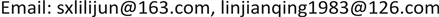1山东师范大学，数学与统计学院，山东 济南

2临沂大学，数学与统计学院，山东 临沂

3朔州师范高等专科学校，数计系，山西 朔州1. 引言

(I)类方程： x ˙ = − y + d x + l x 2 + m x y + n y 2 ,   y ˙ = x 。

(II)类方程： x ˙ = − y + d x + l x 2 + m x y + n y 2 ,   y ˙ = x ( 1 + a x ) ,   a ≠ 0 。

(III)类方程： x ˙ = − y + d x + l x 2 + m x y + n y 2 ,   y ˙ = x ( 1 + a x + b y ) ,   b ≠ 0 。

2. 预备知识

x ˙ = P ( x , y ) ,   y ˙ = Q ( x , y ) (2.1)

F ( θ ) = ∫ 0 θ f ( s ) d s = g θ + φ ( θ ) (2.2)

1) F正定： F ( 0 , 0 ) = 0 ； F ( x , y ) > 0 ，当 ( x , y ) ≠ ( 0 , 0 ) 。

2) d F d t | ( 2.1 ) < 0 ( > 0 ) ，当 ( x , y ) ≠ ( 0 , 0 ) 。

W 1 = m ( l + n ) − a ( b + 2 l ) .

W 2 = m a ( 5 a − m ) [ ( l + n ) 2 ( n + b ) − a 2 ( b + 2 l + n ) ] .

W 3 = m a 2 [ 2 a 2 + n ( l + 2 n ) ] [ ( l + n ) 2 ( n + b ) − a 2 ( b + 2 l + n ) ] .

1) 当 d = 0 ， W 1 ≠ 0 ，则点 O ( 0 , 0 ) 为一阶细焦点， W 1 < 0 时点 O ( 0 , 0 ) 为稳定， W 1 > 0 时点 O ( 0 , 0 ) 为不稳定；

2) 当 d = W 1 = 0 ， W 2 ≠ 0 ，则点 O ( 0 , 0 ) 为二阶细焦点， W 2 < 0 时点 O ( 0 , 0 ) 为稳定， W 2 > 0 时点 O ( 0 , 0 ) 为不稳定；

3) 当 d = W 1 = W 2 = 0 ， W 3 ≠ 0 ，则点 O ( 0 , 0 ) 为三阶细焦点， W 3 < 0 时点 O ( 0 , 0 ) 为稳定， W 3 > 0 时点 O ( 0 , 0 ) 为不稳定；

4) 当 d = W 1 = W 2 = W 3 时，点 O ( 0 , 0 ) 为中心。

3. 主要结果

O ( 0 , 0 ) ,   A ( 0 , − 1 ) ,   B ( − 1 a , 0 ) ,   C ( − 1 a , − 2 ) .

1) 由于 O ( 0 , 0 ) 是对应线性系统的中心，对其非线性系统在原点的性态分析，本文给出三种不同的方法判断原系统在原点的性态，对于在点处的奇点性态，要作一线性变换，把它先移到原点进而再判断其稳定性态。

，至此便可判定原点为原系统的一阶细焦点，当时为不稳定，时为稳定。

2) 接下来我们对进行分析，首先作一线性变换    

，得

3) 同理，对于，作一线性变换，令，得

4) 对于，作一线性变换，令，得

。故而当时原点为不稳定结点；当时原点为鞍点。